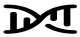# Directed acyclic graph (DAG)

A DAG is a visual representation of potential causal relationships between variables. These relationships are demonstrated by nodes (representing variables) and arrows (a.k.a. edges or arches) between variables. The relationship between two variables in each DAG must be directed (i.e., there cannot be a bidirectional relationship). Relationships between all variables must be acyclic (i.e., a variable cannot have an impact on itself through any number of other variables).

DAGs are useful tools for explicitly demonstrating the underlying assumptions of a proposed analysis. Arrows are drawn between any two variables according to the following criteria: - An arrow from one variable to a second indicates that you assume that it is plausible that the first variable causes the second. - Where there is no arrow between one variable and a second, this indicates that you assume that there is no causal relationship between the first and second variable. Thus, the three key assumptions of IV analyses are illustrated by an arrow from the IV to exposure; an absence of an arrow from the IV to confounders of exposure and outcome; and an absence of an arrow from the IV directly to the outcome.